Complete all Exercises, and submit answers to Questions on the Coursera platform.

## Modeling Wages

In the field of labor economics, the study of income and wages provides insight about topics ranging from gender discrimination to the benefits of higher education. In this lab, we will analyze cross-sectional wage data in order to practice using Bayesian methods such as BIC and Bayesian Model Averaging to construct parsimonious predictive models.

## Getting Started

In this lab we will explore the data using the dplyr package and visualize it using the ggplot2 package for data visualization. We also may use the MASS package to implement stepwise linear regression in one of the exercises. The data can be found in the companion package for this course, statsr.

library(statsr)
library(MASS)
library(dplyr)
library(ggplot2)
library(BAS)
## Warning: package 'BAS' was built under R version 3.4.3

This is the first time we’re using the BAS package. We will be using the bas.lm function from this package later in the lab to implement Bayesian Model Averaging. Please make sure that the version of BAS is 1.3.0 or greater.

### The data

The data will be using in this lab were gathered as a random sample of 935 respondents throughout the United States. This data set was released as part of the series Instructional Stata Datasets for Econometrics by the Boston College Department of Economics (Wooldridge 2000).

data(wage)
variable description
wage weekly earnings (dollars)
hours average hours worked per week
IQ IQ score
kww knowledge of world work score
educ number of years of education
exper years of work experience
tenure years with current employer
age age in years
married =1 if married
black =1 if black
south =1 if live in south
urban =1 if live in a Standard Metropolitan Statistical Area
sibs number of siblings
brthord birth order
meduc mother’s education (years)
feduc father’s education (years)
lwage natural log of wage
1. Is this an observational study or an experiment?
1. Observational study
2. Experiment

## Exploring the data

As with any new data set a good place to start is standard exploratory data analysis. We will begin with the wage variable since it will be the response variable in our models.

1. Which of the following statements is false about the distribution of wage?
1. The median of the distribution is 905.
2. 25% of respondents make more than 1160 dollars per week.
3. 7 of the respondents make less than 300 dollars per week
4. wage is right-skewed, meaning that more respondents fall below the mean wage than above it.
# type your code for Question 2 here, and Knit
summary(wage$wage) ## Min. 1st Qu. Median Mean 3rd Qu. Max. ## 115.0 669.0 905.0 957.9 1160.0 3078.0 quantile(x = wage$wage, probs = c(.07, .25, .50))
##     7%    25%    50%
## 473.14 669.00 905.00
ggplot(wage, aes(x=wage)) +
geom_histogram() +
ggtitle("Histogram of weekly earnings") +
xlab("weekly earnings (dollars)") +
ylab("count")
## stat_bin() using bins = 30. Pick better value with binwidth.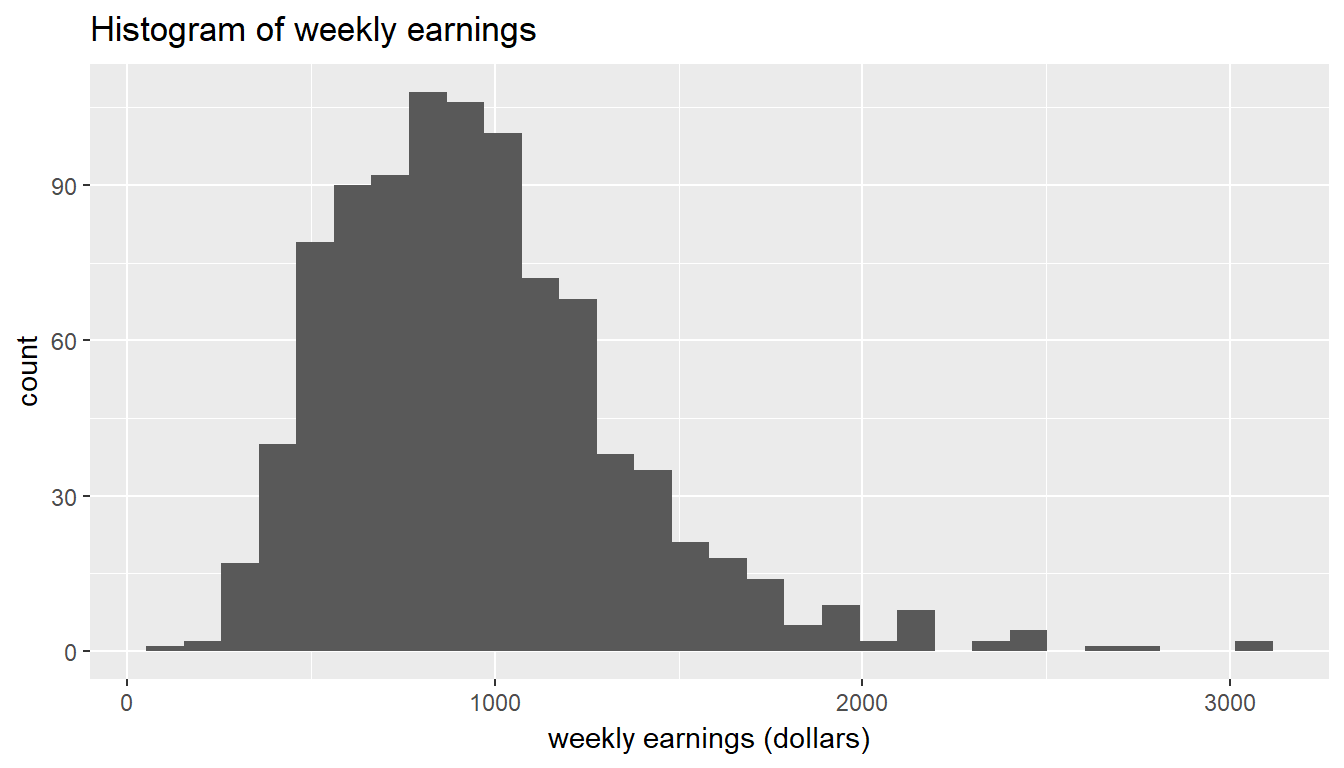Since wage is our response, we would like to explore the relationship of the other variables as predictors.

Exercise: Excluding wage and lwage, select two other variables that you think might be a good predictor of wage. Visualize their relationships with wage using appropriate plots.

# type your code for the Exercise here, and Knit
ols <- lm(wage ~ educ, data = wage)
ggplot(wage, aes(x=educ, y=wage)) +
geom_jitter() +
geom_abline(intercept = ols$coefficients, slope = ols$coefficients) +
ggtitle("wages vs. education") +
xlab("number of years of education") +
ylab("weekly earnings (dollars)")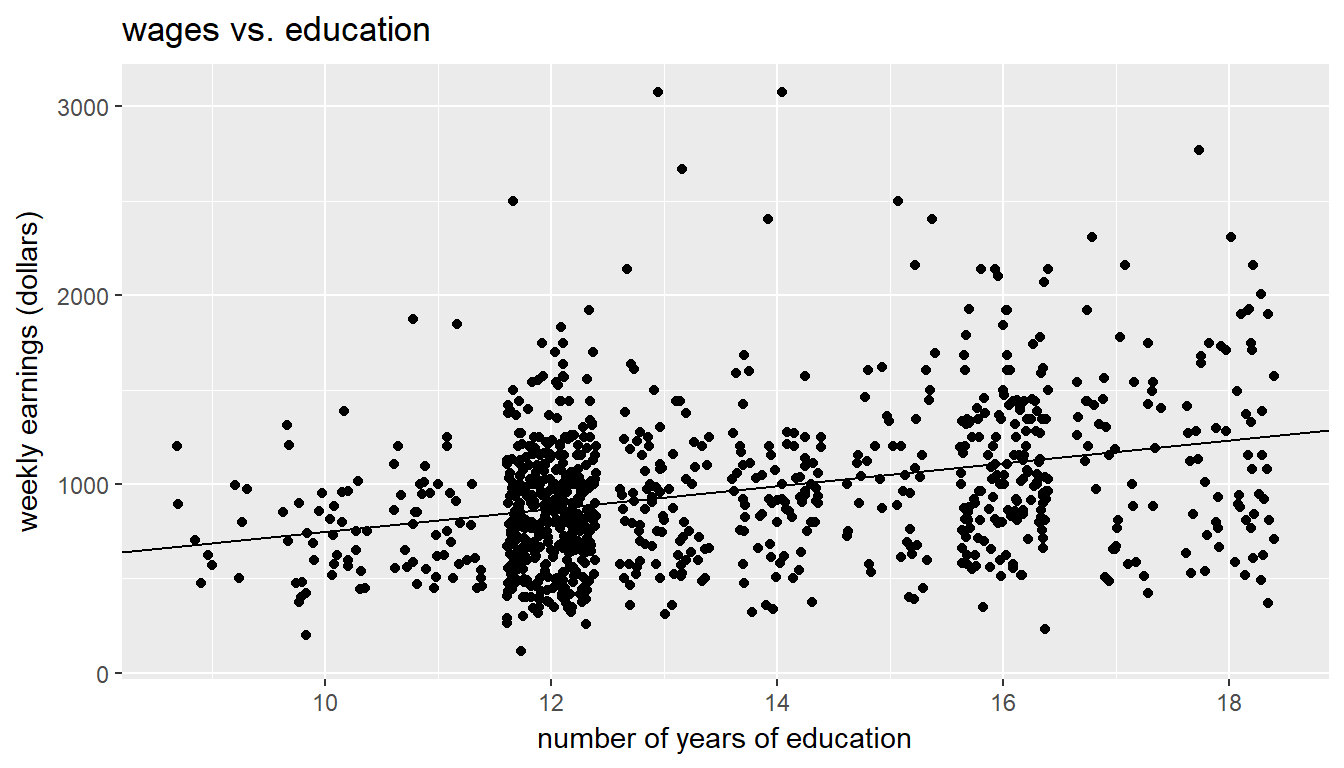ols <- lm(wage ~ exper, data = wage)
ggplot(wage, aes(x=exper, y=wage)) +
geom_jitter() +
geom_abline(intercept = ols$coefficients, slope = ols$coefficients) +
ggtitle("wages vs. experience") +
xlab("years of work experience") +
ylab("weekly earnings (dollars)")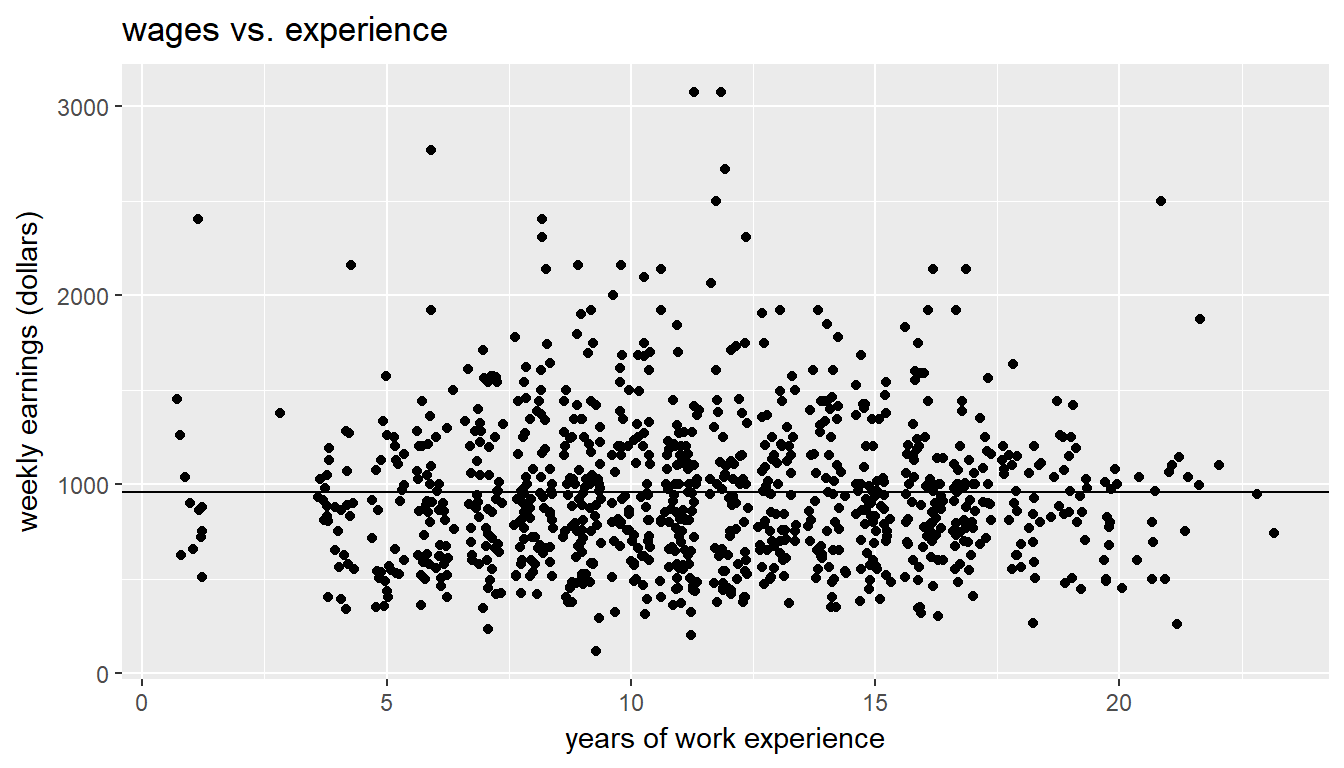## Simple linear regression

One possible, simplistic, explanation for the variation in wages that we see in the data is that smarter people make more money. The plot below visualizes a scatterplot between weekly wage and IQ score.

ggplot(data = wage, aes(x = iq, y = wage)) +
geom_point()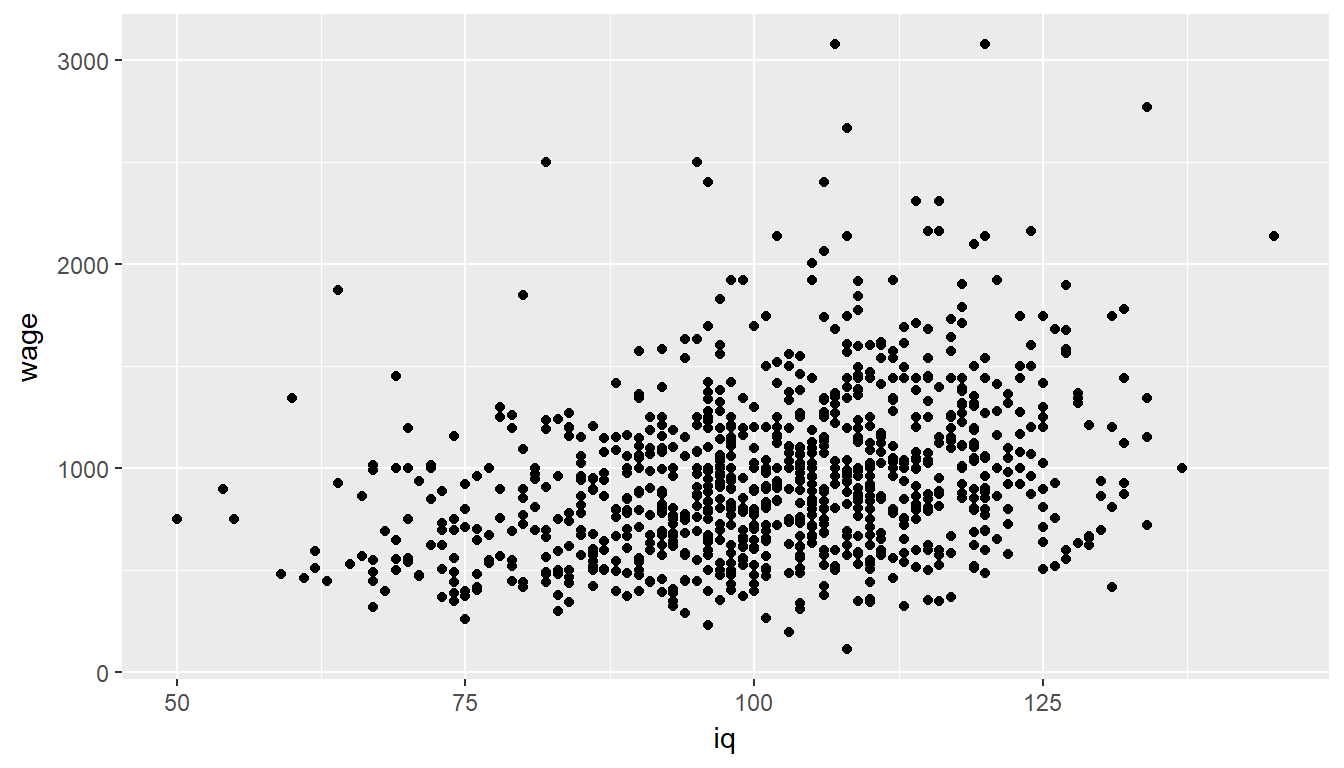This plot is rather noisy. While there may be a slight positive linear relationship between IQ score and wage, IQ is at best a crude predictor of wages. We can quantify this by fitting a simple linear regression.

m_wage_iq = lm(wage ~ iq, data = wage)
m_wage_iq$coefficients ## (Intercept) iq ## 116.991565 8.303064 summary(m_wage_iq)$sigma
##  384.7667

Recall from the lectures that under the model

$wage_i = \alpha + \beta \cdot iq_i + \epsilon_i$

if $$\epsilon_i \sim N(0, \sigma^2)$$ and the reference prior $$p(\alpha, \beta, \sigma^2) \propto 1/\sigma^2$$ is used, then the Bayesian posterior means and standard deviations will be equal to the frequentist estimates and standard errors respectively.

The Bayesian model specification assumes that the errors are normally distributed with a constant variance. As with the frequentist approach we check this assumption by examining the distribution of the residuals for the model. If the residuals are highly non-normal or skewed, the assumption is violated and any subsequent inference is not valid.

1. Examine the residuals of m_wage_iq. Is the assumption of normally distributed errors valid?
1. Yes, since the distribution of the dependent variable (wage) is roughly normally distributed.
2. Yes, since the distribution of the residuals of the model looks approximately normal.
3. No, since the distribution of the residuals of the model is left-skewed.
4. No, since the distribution of the residuals of the model is right-skewed.
# type your code for Question 3 here, and Knit
# A plot of residuals vs the explanatory variable reveals independence.
# A plot of residuals vs the fitted variable reveals homogeneity.
# A histogram of residuals reveals normality.  Or use a normal probability plot (qqnorm)
m_wage_iq.resid = resid(m_wage_iq)
m_wage_iq.stdres = rstandard(m_wage_iq)
qqnorm(m_wage_iq.stdres,
xlab = "Standardized Residuals",
ylab = "Normal Scores",
main = "Normal Probability Plot of Residuals")
qqline(m_wage_iq.stdres)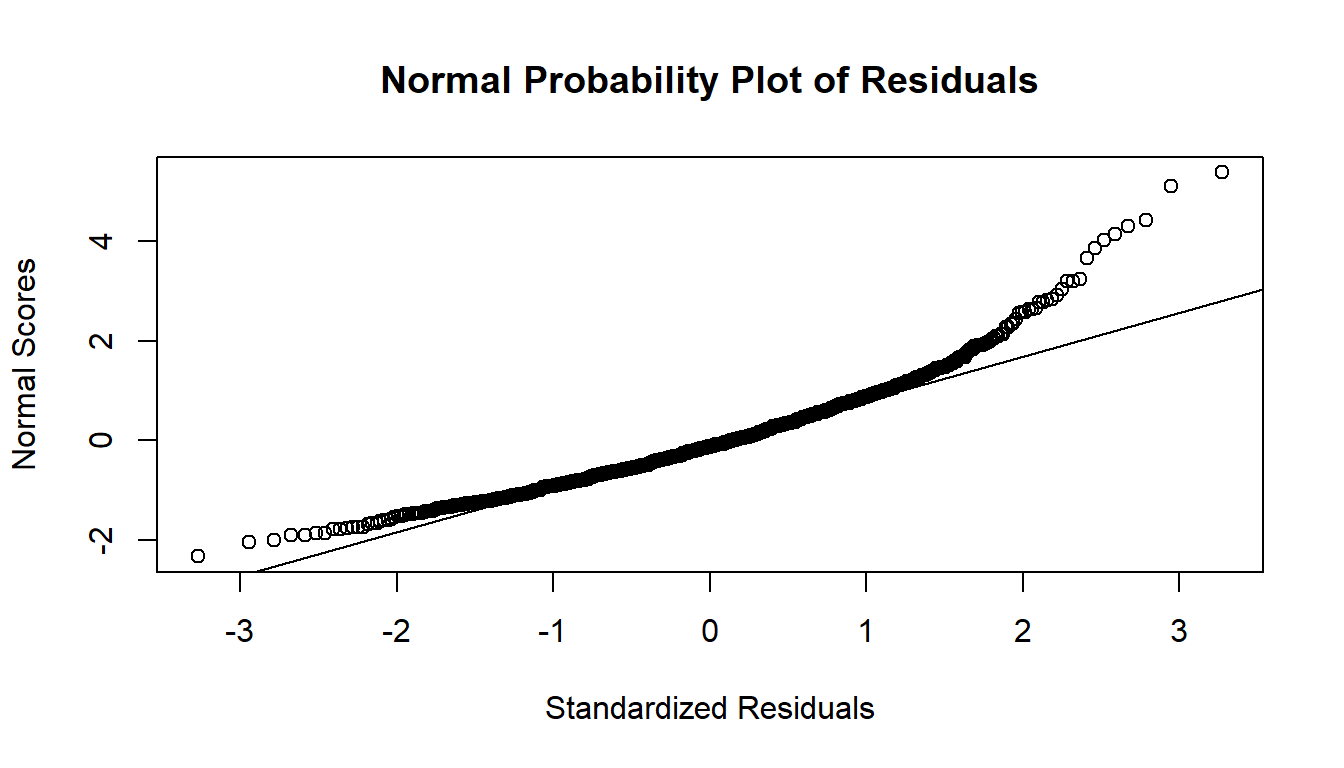ggplot(wage, aes(x=m_wage_iq.stdres)) +
geom_histogram()
## stat_bin() using bins = 30. Pick better value with binwidth.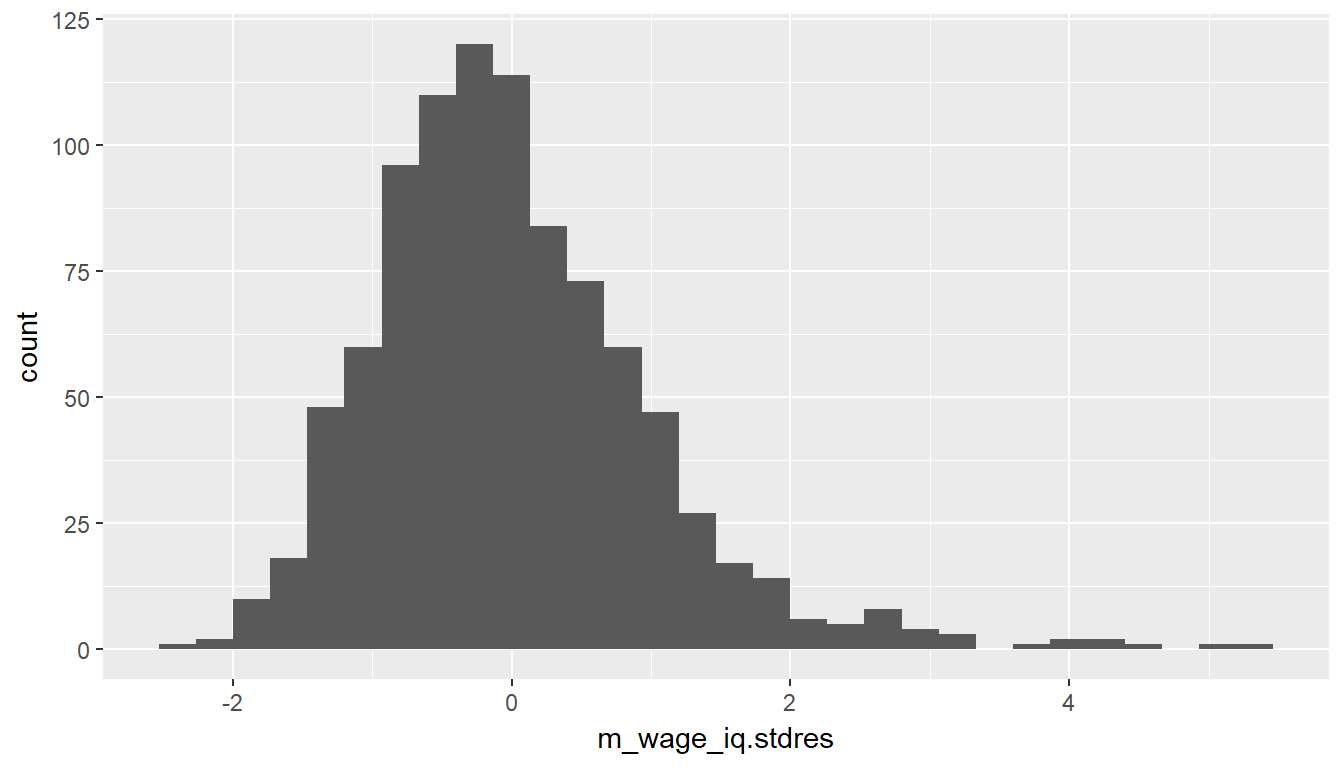Exercise: Refit the model, this time using educ (education) as the independent variable. Does your answer to the previous exercise change?

# type your code for the Exercise here, and Knit
m_wage_educ <- lm(wage ~ educ, data = wage)
m_wage_educ.stdres = rstandard(m_wage_educ)
qqnorm(m_wage_educ.stdres,
xlab = "Standardized Residuals",
ylab = "Normal Scores",
main = "Normal Probability Plot of Residuals")
qqline(m_wage_educ.stdres)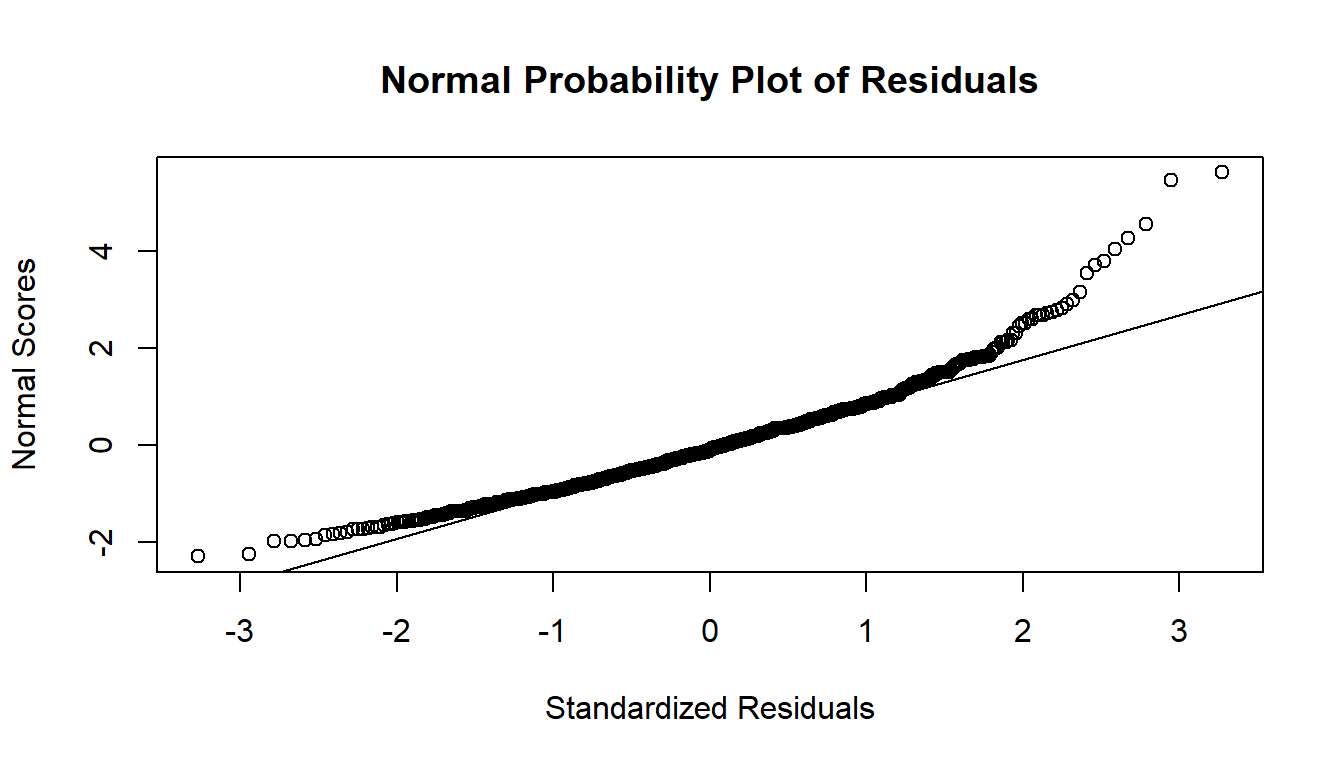ggplot(wage, aes(x=m_wage_educ.stdres)) +
geom_histogram()
## stat_bin() using bins = 30. Pick better value with binwidth.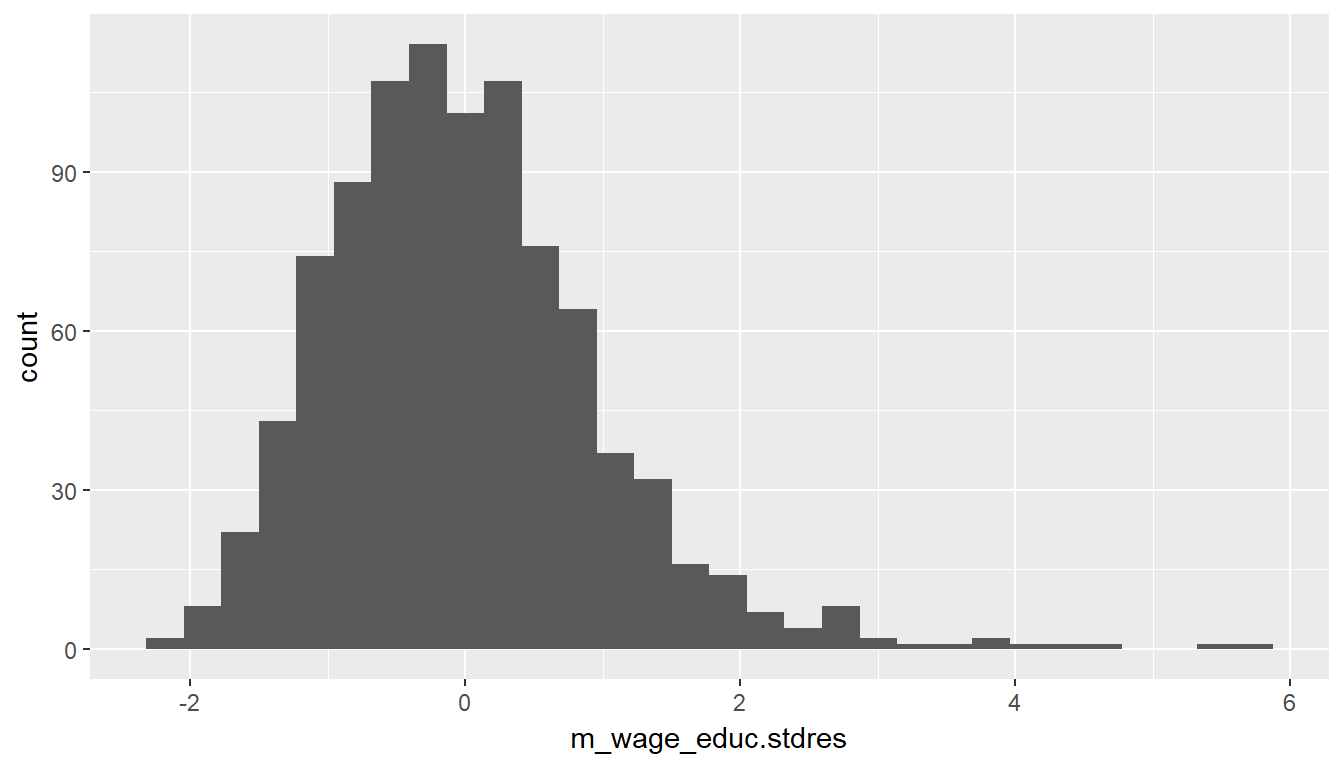## Variable transformation

One way to accommodate the right-skewness in the data is to (natural) log transform the dependent variable. Note that this is only possible if the variable is strictly positive, since the log of negative value is not defined and $$\log(0) = -\infty$$. Let’s try to fit a linear model with log-wage as the dependent variable. Question 4 will be based on this log transformed model.

m_lwage_iq = lm(lwage ~ iq, data = wage)
m_lwage_iq$coefficients ## (Intercept) iq ## 5.886994223 0.008807157 summary(m_lwage_iq)$sigma
##  0.399948
summary(m_lwage_iq)
##
## Call:
## lm(formula = lwage ~ iq, data = wage)
##
## Residuals:
##      Min       1Q   Median       3Q      Max
## -2.09324 -0.25547  0.02261  0.27544  1.21486
##
## Coefficients:
##              Estimate Std. Error t value Pr(>|t|)
## (Intercept) 5.8869942  0.0890206   66.13   <2e-16 ***
## iq          0.0088072  0.0008694   10.13   <2e-16 ***
## ---
## Signif. codes:  0 '***' 0.001 '**' 0.01 '*' 0.05 '.' 0.1 ' ' 1
##
## Residual standard error: 0.3999 on 933 degrees of freedom
## Multiple R-squared:  0.09909,    Adjusted R-squared:  0.09813
## F-statistic: 102.6 on 1 and 933 DF,  p-value: < 2.2e-16

Exercise: Examine the residuals of this model. Is the assumption of normally distributed residuals reasonable?

# type your code for the Exercise here, and Knit
m_lwage_iq.stdres = rstandard(m_lwage_iq)
qqnorm(m_lwage_iq.stdres,
xlab = "Standardized Residuals",
ylab = "Normal Scores",
main = "Normal Probability Plot of Residuals")
qqline(m_lwage_iq.stdres)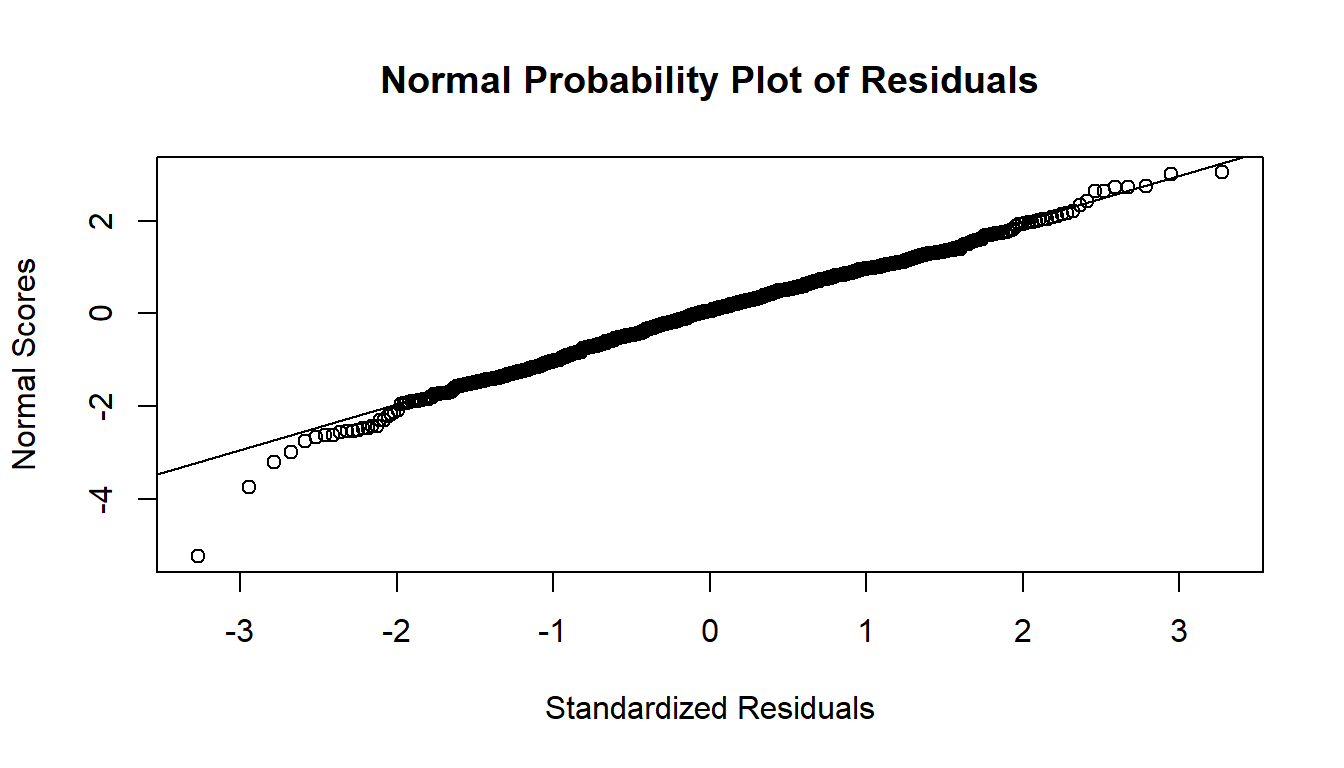ggplot(wage, aes(x=m_lwage_iq.stdres)) +
geom_histogram()
## stat_bin() using bins = 30. Pick better value with binwidth.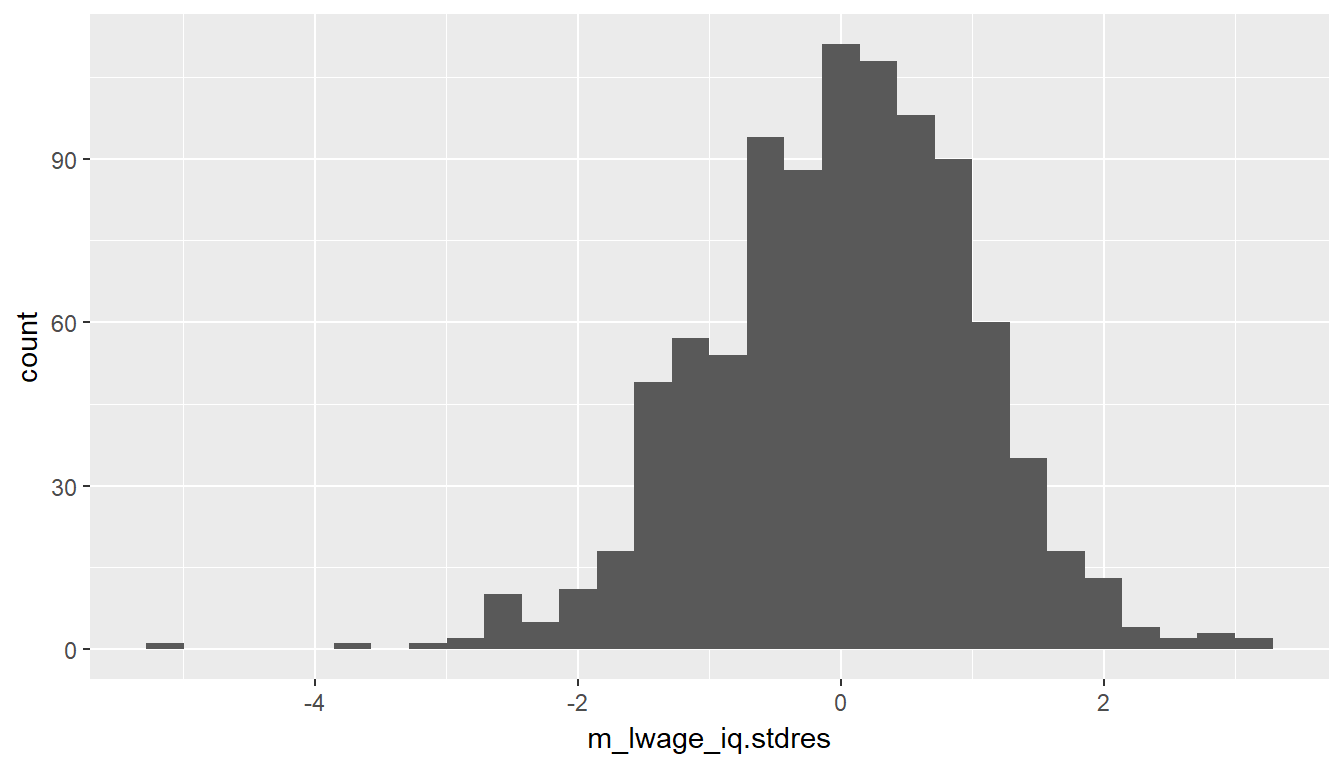Recall that the posterior distribution of $$\alpha$$ and $$\beta$$ given $$\sigma^2$$ is normal, but marginally follows a $$t$$ distribution with $$n-p-1$$ degrees of freedom. In this case, $$p=1$$, since IQ is the only predictor of log-wage included in our model. Therefore both $$\alpha$$ and $$\beta$$ will have posteriors that follow a $$t$$ distribution 933 degrees of freedom - since the df is so large these distributions will actually be approximately normal.

1. Under the reference prior $$p(\alpha, \beta, \sigma^2) \propto 1/\sigma^2$$, give a 95% posterior credible interval for $$\beta$$, the coefficient of IQ.
1. (0.00793, 0.00967)
2. (0.00709, 0.01050)
3. (0.00663, 0.01098)
4. (0.00010, 0.01750)
# type your code for Question 4 here, and Knit
summary(m_lwage_iq)$coefficients - qt(.975,933)*summary(m_lwage_iq)$coefficients
##  0.007100959
summary(m_lwage_iq)$coefficients + qt(.975,933)*summary(m_lwage_iq)$coefficients
##  0.01051335

Exercise: The coefficient of IQ is very small, which is expected since a one point increase in IQ score can hardly be expected to have a high multiplicative effect on wage. One way to make the coefficient more interpretable is to standardize IQ before putting it into the model. From this new model, an increase in IQ of 1 standard deviation (15 points) is estimated to increase wage by what percentage?

# type your code for the Exercise here, and Knit
wage <- wage %>% mutate(siq = iq / 15)
m_lwage_siq <- lm(lwage ~ siq, data = wage)
summary(m_lwage_siq)\$coefficients
##  0.1321074

# Multiple linear regression

It is evident that wage can be explained by many predictors, such as experience, education, and IQ. We can include all relevant covariates in a regression model in an attempt to explain as much wage variation as possible.

m_lwage_full = lm(lwage ~ . - wage, data = wage)

The use of . in the lm tells R to include all covariates in the model which we then further modify with -wage which then excludes the wage variable from the model.

However, running this full model has a cost: we remove observations from our data since some measurements for (e.g. birth order, mother’s education, and father’s education) are missing. By default, the lm function does a complete-case analysis, and so it removes any observations with a missing (NA) value in one or more of the predictor variables.

Because of these missing values we must make an additional assumption for our inferences to be valid. This exclusion of rows with missing values requires that there is no systematic reason for the values to be missing, or in other words our data must be missing at random. For example, if all first-born children did not report their birth order, the data would not be missing at random. Without any additional information we will assume this is reasonable and use the 663 complete observations (as opposed to the original 935) to fit the model. Both Bayesian and frequentist methods exist to handle data sets with missing data, but they are beyond the scope of this course.

1. From the model, all else begin equal, who would you expect to make more: a married black man or a single non-black man?
1. The married black man
2. The single non-black man
# type your code for Question 5 here, and Knit
summary(m_lwage_full)
##
## Call:
## lm(formula = lwage ~ . - wage, data = wage)
##
## Residuals:
##      Min       1Q   Median       3Q      Max
## -1.96887 -0.19460  0.00923  0.22401  1.34185
##
## Coefficients: (1 not defined because of singularities)
##              Estimate Std. Error t value Pr(>|t|)
## (Intercept)  5.156439   0.225286  22.888  < 2e-16 ***
## hours       -0.006548   0.001934  -3.385 0.000754 ***
## iq           0.003186   0.001223   2.604 0.009425 **
## kww          0.003735   0.002390   1.562 0.118662
## educ         0.041267   0.008942   4.615 4.74e-06 ***
## exper        0.010749   0.004435   2.424 0.015629 *
## tenure       0.007102   0.002894   2.454 0.014401 *
## age          0.009107   0.005977   1.524 0.128058
## married1     0.200760   0.045998   4.365 1.48e-05 ***
## black1      -0.105141   0.055667  -1.889 0.059373 .
## south1      -0.049076   0.030753  -1.596 0.111019
## urban1       0.195658   0.031240   6.263 6.88e-10 ***
## sibs         0.009619   0.007876   1.221 0.222423
## brthord     -0.018465   0.011569  -1.596 0.110975
## meduc        0.009633   0.006167   1.562 0.118753
## feduc        0.005590   0.005398   1.036 0.300805
## siq                NA         NA      NA       NA
## ---
## Signif. codes:  0 '***' 0.001 '**' 0.01 '*' 0.05 '.' 0.1 ' ' 1
##
## Residual standard error: 0.3507 on 647 degrees of freedom
##   (272 observations deleted due to missingness)
## Multiple R-squared:  0.2925, Adjusted R-squared:  0.2761
## F-statistic: 17.84 on 15 and 647 DF,  p-value: < 2.2e-16

As you can see from a quick summary of the full linear model, many coefficients of independent variables are not statistically significant. In previous labs within this specialization, you selected variables based on Adjusted $$R^2.$$ This module introduced the Bayesian Information Criterion (BIC), which is a metric that can be used for model selection. BIC is based on model fit, while simultaneously penalizing the number of parameters in proportion to the sample size. We can calculate the BIC of the full linear model using the command below:

BIC(m_lwage_full)
##  586.3732

We can compare the BIC of the full model with that of a reduced model. Let’s try to remove birth order from the model. To ensure that the observations remain the same, the data set can be specified as na.omit(wage), which includes only the observations with no missing values.

m_lwage_nobrthord = lm(lwage ~ . -wage -brthord, data = na.omit(wage))
BIC(m_lwage_nobrthord)
##  582.4815

As you can see, removing birth order from the regression reduces BIC, which we seek to minimize by model selection.

1. Elimination of which variable from the full model yielded the lowest BIC?
1. brthord
2. sibs
3. feduc
4. meduc
# type your code for Question 6 here, and Knit
m_lwage_nobrthord = lm(lwage ~ . -wage -brthord, data = na.omit(wage))
BIC(m_lwage_nobrthord)
##  582.4815
m_lwage_nosibs = lm(lwage ~ . -wage -sibs, data = na.omit(wage))
BIC(m_lwage_nosibs)
##  581.4031
m_lwage_nofeduc = lm(lwage ~ . -wage -feduc, data = na.omit(wage))
BIC(m_lwage_nofeduc)
##  580.9743
m_lwage_nomeduc = lm(lwage ~ . -wage -meduc, data = na.omit(wage))
BIC(m_lwage_nomeduc)
##  582.3722

Exercise: R has a function stepAIC that will work backwards through the model space, removing variables until BIC can be no longer be lowered. It takes as inputs a full model, and a penalty parameter $$k$$. Find the best model according to BIC (in which case $$k = \log(n)$$). Remember to use na.omit(wage) as your data set.

# type your code for the Exercise here, and Knit
m_lwage_full = lm(lwage ~ . - wage, data = na.omit(wage))
stepAIC(m_lwage_full, k = log(nrow(na.omit(wage))))
## Start:  AIC=-1301.64
## lwage ~ (wage + hours + iq + kww + educ + exper + tenure + age +
##     married + black + south + urban + sibs + brthord + meduc +
##     feduc + siq) - wage
##
##
## Step:  AIC=-1301.64
## lwage ~ hours + iq + kww + educ + exper + tenure + age + married +
##     black + south + urban + sibs + brthord + meduc + feduc
##
##           Df Sum of Sq    RSS     AIC
## - feduc    1    0.1319 79.710 -1307.0
## - sibs     1    0.1835 79.761 -1306.6
## - age      1    0.2856 79.864 -1305.8
## - meduc    1    0.3001 79.878 -1305.6
## - kww      1    0.3003 79.878 -1305.6
## - south    1    0.3132 79.891 -1305.5
## - brthord  1    0.3133 79.891 -1305.5
## - black    1    0.4388 80.017 -1304.5
## - exper    1    0.7226 80.301 -1302.1
## - tenure   1    0.7405 80.319 -1302.0
## <none>                 79.578 -1301.6
## - iq       1    0.8340 80.412 -1301.2
## - hours    1    1.4096 80.988 -1296.5
## - married  1    2.3430 81.921 -1288.9
## - educ     1    2.6196 82.198 -1286.7
## - urban    1    4.8246 84.403 -1269.1
##
## Step:  AIC=-1307.03
## lwage ~ hours + iq + kww + educ + exper + tenure + age + married +
##     black + south + urban + sibs + brthord + meduc
##
##           Df Sum of Sq    RSS     AIC
## - sibs     1    0.1948 79.905 -1311.9
## - age      1    0.2594 79.969 -1311.4
## - kww      1    0.3152 80.025 -1310.9
## - brthord  1    0.3386 80.049 -1310.7
## - south    1    0.3448 80.055 -1310.7
## - black    1    0.4730 80.183 -1309.6
## - meduc    1    0.6491 80.359 -1308.2
## - exper    1    0.6991 80.409 -1307.7
## - tenure   1    0.7271 80.437 -1307.5
## <none>                 79.710 -1307.0
## - iq       1    0.8521 80.562 -1306.5
## - hours    1    1.4168 81.127 -1301.8
## - married  1    2.3525 82.062 -1294.2
## - educ     1    2.9193 82.629 -1289.7
## - urban    1    4.9771 84.687 -1273.4
##
## Step:  AIC=-1311.91
## lwage ~ hours + iq + kww + educ + exper + tenure + age + married +
##     black + south + urban + brthord + meduc
##
##           Df Sum of Sq    RSS     AIC
## - brthord  1    0.1663 80.071 -1317.0
## - kww      1    0.2676 80.172 -1316.2
## - age      1    0.2832 80.188 -1316.1
## - black    1    0.3724 80.277 -1315.3
## - south    1    0.4023 80.307 -1315.1
## - meduc    1    0.6089 80.514 -1313.4
## - exper    1    0.6546 80.559 -1313.0
## - tenure   1    0.7133 80.618 -1312.5
## <none>                 79.905 -1311.9
## - iq       1    0.8310 80.736 -1311.5
## - hours    1    1.3930 81.298 -1307.0
## - married  1    2.3932 82.298 -1298.8
## - educ     1    2.8678 82.773 -1295.0
## - urban    1    4.8999 84.805 -1279.0
##
## Step:  AIC=-1317.03
## lwage ~ hours + iq + kww + educ + exper + tenure + age + married +
##     black + south + urban + meduc
##
##           Df Sum of Sq    RSS     AIC
## - kww      1    0.2743 80.345 -1321.3
## - age      1    0.2854 80.356 -1321.2
## - black    1    0.3883 80.459 -1320.3
## - south    1    0.4449 80.516 -1319.8
## - exper    1    0.6721 80.743 -1318.0
## - tenure   1    0.7084 80.779 -1317.7
## - meduc    1    0.7827 80.854 -1317.1
## <none>                 80.071 -1317.0
## - iq       1    0.8853 80.956 -1316.2
## - hours    1    1.3401 81.411 -1312.5
## - married  1    2.4160 82.487 -1303.8
## - educ     1    2.9179 82.989 -1299.8
## - urban    1    4.8863 84.957 -1284.3
##
## Step:  AIC=-1321.26
## lwage ~ hours + iq + educ + exper + tenure + age + married +
##     black + south + urban + meduc
##
##           Df Sum of Sq    RSS     AIC
## - south    1    0.4017 80.747 -1324.5
## - black    1    0.4771 80.822 -1323.8
## - age      1    0.6464 80.992 -1322.5
## - exper    1    0.6572 81.003 -1322.4
## - tenure   1    0.7851 81.130 -1321.3
## <none>                 80.345 -1321.3
## - meduc    1    0.8825 81.228 -1320.5
## - iq       1    1.2318 81.577 -1317.7
## - hours    1    1.2648 81.610 -1317.4
## - married  1    2.4969 82.842 -1307.5
## - educ     1    3.3468 83.692 -1300.7
## - urban    1    5.2282 85.574 -1286.0
##
## Step:  AIC=-1324.45
## lwage ~ hours + iq + educ + exper + tenure + age + married +
##     black + urban + meduc
##
##           Df Sum of Sq    RSS     AIC
## - black    1    0.6104 81.357 -1326.0
## - exper    1    0.6681 81.415 -1325.5
## - age      1    0.6962 81.443 -1325.3
## <none>                 80.747 -1324.5
## - tenure   1    0.8571 81.604 -1324.0
## - meduc    1    1.0008 81.748 -1322.8
## - hours    1    1.2409 81.988 -1320.8
## - iq       1    1.3912 82.138 -1319.6
## - married  1    2.4655 83.212 -1311.0
## - educ     1    3.2391 83.986 -1304.9
## - urban    1    5.6789 86.426 -1285.9
##
## Step:  AIC=-1325.96
## lwage ~ hours + iq + educ + exper + tenure + age + married +
##     urban + meduc
##
##           Df Sum of Sq    RSS     AIC
## - exper    1    0.6402 81.998 -1327.3
## <none>                 81.357 -1326.0
## - age      1    0.8029 82.160 -1325.9
## - tenure   1    0.8925 82.250 -1325.2
## - hours    1    1.1672 82.525 -1323.0
## - meduc    1    1.2197 82.577 -1322.6
## - iq       1    2.1364 83.494 -1315.3
## - married  1    2.5715 83.929 -1311.8
## - educ     1    3.0124 84.370 -1308.3
## - urban    1    5.3797 86.737 -1290.0
##
## Step:  AIC=-1327.26
## lwage ~ hours + iq + educ + tenure + age + married + urban +
##     meduc
##
##           Df Sum of Sq    RSS     AIC
## <none>                 81.998 -1327.3
## - meduc    1    1.2031 83.201 -1324.1
## - tenure   1    1.2280 83.226 -1323.9
## - hours    1    1.3140 83.312 -1323.2
## - iq       1    2.2622 84.260 -1315.7
## - age      1    2.3323 84.330 -1315.2
## - educ     1    2.3730 84.371 -1314.8
## - married  1    2.6676 84.665 -1312.5
## - urban    1    5.4901 87.488 -1290.8
##
## Call:
## lm(formula = lwage ~ hours + iq + educ + tenure + age + married +
##     urban + meduc, data = na.omit(wage))
##
## Coefficients:
## (Intercept)        hours           iq         educ       tenure
##    4.888461    -0.006268     0.004821     0.033262     0.008942
##         age     married1       urban1        meduc
##    0.020354     0.213519     0.203908     0.016442

## Bayesian model averaging

Often, several models are equally plausible and choosing only one ignores the inherent uncertainty involved in choosing the variables to include in the model. A way to get around this problem is to implement Bayesian model averaging (BMA), in which multiple models are averaged to obtain posteriors of coefficients and predictions from new data. Dr. Merlise Clyde is the primary author of the R package BAS, which implements BMA. We can use this for either implementing BMA or selecting models. We start by applying BMA to the wage data.

wage_no_na = na.omit(wage)
bma_lwage = bas.lm(lwage ~ . -wage, data = wage_no_na,
prior = "BIC",
modelprior = uniform())
bma_lwage
##
## Call:
## bas.lm(formula = lwage ~ . - wage, data = wage_no_na, prior = "BIC",     modelprior = uniform())
##
##
##  Marginal Posterior Inclusion Probabilities:
## Intercept      hours         iq        kww       educ      exper
##  1.000000   0.002177   1.000000   0.358252   0.999972   0.914579
##    tenure        age   married1     black1     south1     urban1
##  0.872293   0.355849   0.999796   0.252813   0.404206   1.000000
##      sibs    brthord      meduc      feduc        siq
##  0.040877   0.093574   0.617487   0.331468   1.000000
summary(bma_lwage)
##           P(B != 0 | Y)    model 1       model 2       model 3
## Intercept   1.000000000     1.0000     1.0000000     1.0000000
## hours       0.002176816     0.0000     0.0000000     0.0000000
## iq          1.000000000     1.0000     1.0000000     1.0000000
## kww         0.358252241     0.0000     0.0000000     1.0000000
## educ        0.999971793     1.0000     1.0000000     1.0000000
## exper       0.914579170     1.0000     1.0000000     1.0000000
## tenure      0.872292917     1.0000     1.0000000     1.0000000
## age         0.355849221     0.0000     0.0000000     0.0000000
## married1    0.999795530     1.0000     1.0000000     1.0000000
## black1      0.252812588     0.0000     0.0000000     0.0000000
## south1      0.404206273     0.0000     1.0000000     1.0000000
## urban1      0.999999993     1.0000     1.0000000     1.0000000
## sibs        0.040876562     0.0000     0.0000000     0.0000000
## brthord     0.093573589     0.0000     0.0000000     0.0000000
## meduc       0.617486851     1.0000     1.0000000     1.0000000
## feduc       0.331468049     0.0000     0.0000000     0.0000000
## siq         1.000000000     1.0000     1.0000000     1.0000000
## BF                   NA     1.0000     0.7044423     0.6935815
## PostProbs            NA     0.0718     0.0505000     0.0498000
## R2                   NA     0.3936     0.3988000     0.4047000
## dim                  NA     9.0000    10.0000000    11.0000000
## logmarg              NA -1429.0488 -1429.3991632 -1429.4147009
##                 model 4       model 5
## Intercept     1.0000000     1.0000000
## hours         0.0000000     0.0000000
## iq            1.0000000     1.0000000
## kww           1.0000000     0.0000000
## educ          1.0000000     1.0000000
## exper         1.0000000     1.0000000
## tenure        1.0000000     1.0000000
## age           0.0000000     1.0000000
## married1      1.0000000     1.0000000
## black1        0.0000000     0.0000000
## south1        0.0000000     0.0000000
## urban1        1.0000000     1.0000000
## sibs          0.0000000     0.0000000
## brthord       0.0000000     0.0000000
## meduc         1.0000000     1.0000000
## feduc         0.0000000     0.0000000
## siq           1.0000000     1.0000000
## BF            0.6044052     0.5186109
## PostProbs     0.0434000     0.0372000
## R2            0.3986000     0.3983000
## dim          10.0000000    10.0000000
## logmarg   -1429.5523248 -1429.7054157

Printing the model object and the summary command gives us both the posterior model inclusion probability for each variable and the most probable models. For example, the posterior probability that hours is included in the model is 0.855. Further, the most likely model, which has posterior probability of 0.0455, includes an intercept, hours worked, IQ, education, tenure, age, marital status, urban living status, and mother’s education. While a posterior probability of 0.0455 sounds small, it is much larger than the uniform prior probability assigned to it, since there are $$2^{16}$$ possible models.

It is also possible to visualize the posterior distribution of the coefficients under the model averaging approach. We graph the posterior distribution of the coefficients of iq and sibs below. Note that the subset command dictates which variable is plotted.

par(mfrow = c(1,2))
coef_lwage = coefficients(bma_lwage)
plot(coef_lwage, subset = c(3,13), ask=FALSE)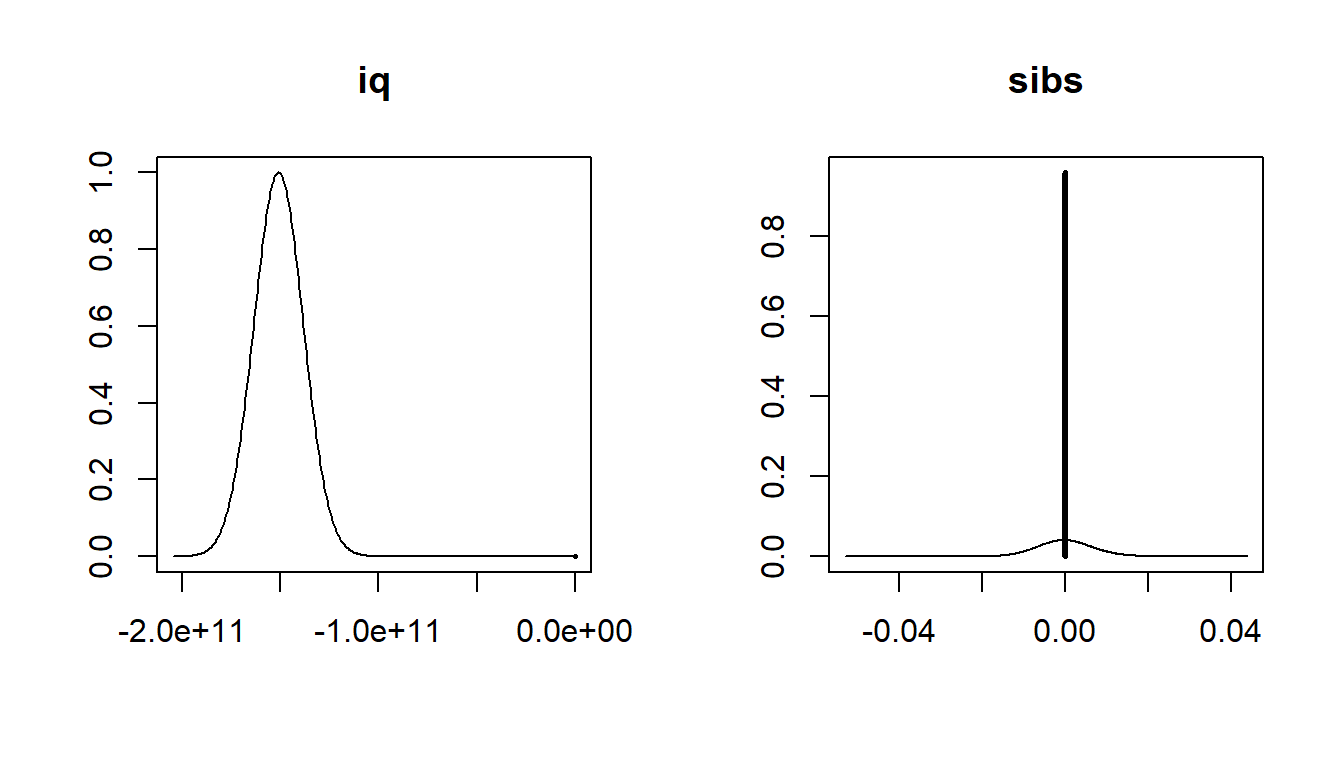We can also provide 95% credible intervals for these coefficients:

confint(coef_lwage)
##                    2.5%         97.5%          beta
## Intercept  6.794712e+00  6.843612e+00  6.819200e+00
## hours      0.000000e+00  0.000000e+00 -1.342763e-05
## iq        -1.753708e+11 -1.260719e+11 -1.509867e+11
## kww        0.000000e+00  7.299549e-03  1.746289e-03
## educ       2.959239e-02  6.666032e-02  4.771453e-02
## exper      0.000000e+00  2.181320e-02  1.387225e-02
## tenure    -3.757841e-07  1.288894e-02  7.536446e-03
## age       -1.866496e-07  2.044491e-02  4.857477e-03
## married1   1.228078e-01  2.877170e-01  2.047447e-01
## black1    -1.482694e-01  0.000000e+00 -2.638930e-02
## south1    -1.005750e-01  0.000000e+00 -2.660446e-02
## urban1     1.367004e-01  2.498895e-01  1.933523e-01
## sibs       0.000000e+00  0.000000e+00  5.082076e-06
## brthord   -1.445485e-02  3.102682e-06 -1.209797e-03
## meduc      0.000000e+00  2.194590e-02  9.063805e-03
## feduc      0.000000e+00  1.674232e-02  3.774019e-03
## siq        1.895188e+12  2.633433e+12  2.264801e+12
## attr(,"Probability")
##  0.95
## attr(,"class")
##  "confint.bas"

For questions 7-8, we’ll use a reduced data set which excludes number of siblings, birth order, and parental education.

wage_red = wage %>%
select(-sibs, -brthord, -meduc, -feduc)
1. Based on this reduced data set, according to Bayesian model averaging, which of the following variables has the lowest marginal posterior inclusion probability?
1. kww
2. black
3. south
4. age
# type your code for Question 7 here, and Knit
bma_lwage = bas.lm(lwage ~ . -wage, data = wage_red,
prior = "BIC",
modelprior = uniform())
bma_lwage
##
## Call:
## bas.lm(formula = lwage ~ . - wage, data = wage_red, prior = "BIC",     modelprior = uniform())
##
##
##  Marginal Posterior Inclusion Probabilities:
## Intercept      hours         iq        kww       educ      exper
## 1.000e+00  5.732e-09  1.000e+00  2.067e-01  1.000e+00  9.850e-01
##    tenure        age   married1     black1     south1     urban1
## 9.998e-01  2.269e-01  1.000e+00  9.565e-01  9.313e-01  1.000e+00
##       siq
## 1.000e+00
summary(bma_lwage)
##           P(B != 0 | Y)    model 1       model 2       model 3
## Intercept  1.000000e+00     1.0000     1.0000000     1.0000000
## hours      5.731948e-09     0.0000     0.0000000     0.0000000
## iq         1.000000e+00     1.0000     1.0000000     1.0000000
## kww        2.066741e-01     0.0000     0.0000000     1.0000000
## educ       1.000000e+00     1.0000     1.0000000     1.0000000
## exper      9.850360e-01     1.0000     1.0000000     1.0000000
## tenure     9.998193e-01     1.0000     1.0000000     1.0000000
## age        2.269472e-01     0.0000     1.0000000     0.0000000
## married1   9.999901e-01     1.0000     1.0000000     1.0000000
## black1     9.564507e-01     1.0000     1.0000000     1.0000000
## south1     9.313134e-01     1.0000     1.0000000     1.0000000
## urban1     1.000000e+00     1.0000     1.0000000     1.0000000
## siq        1.000000e+00     1.0000     1.0000000     1.0000000
## BF                   NA     1.0000     0.3448479     0.2567238
## PostProbs            NA     0.5369     0.1852000     0.1378000
## R2                   NA     0.3995     0.4025000     0.4021000
## dim                  NA    10.0000    11.0000000    11.0000000
## logmarg              NA -2184.6868 -2185.7514398 -2186.0465424
##                 model 4       model 5
## Intercept  1.000000e+00  1.000000e+00
## hours      0.000000e+00  0.000000e+00
## iq         1.000000e+00  1.000000e+00
## kww        0.000000e+00  1.000000e+00
## educ       1.000000e+00  1.000000e+00
## exper      1.000000e+00  1.000000e+00
## tenure     1.000000e+00  1.000000e+00
## age        0.000000e+00  0.000000e+00
## married1   1.000000e+00  1.000000e+00
## black1     1.000000e+00  0.000000e+00
## south1     0.000000e+00  1.000000e+00
## urban1     1.000000e+00  1.000000e+00
## siq        1.000000e+00  1.000000e+00
## BF         6.671359e-02  5.350752e-02
## PostProbs  3.580000e-02  2.870000e-02
## R2         3.916000e-01  3.957000e-01
## dim        9.000000e+00  1.000000e+01
## logmarg   -2.187394e+03 -2.187615e+03
1. True or False: The naive model with all variables included has posterior probability greater than 0.5. (Use a Zellner-Siow null prior for the coefficients and a Beta-Binomial (1,1) prior for the models)
1. True
2. False
# type your code for Question 8 here, and Knit
bma_lwage = bas.lm(lwage ~ . -wage, data = wage_red,
prior = "ZS-null",
modelprior = beta.binomial(1, 1))
## Warning in bas.lm(lwage ~ . - wage, data = wage_red, prior = "ZS-null", :
## We recommend using the implementation using the Jeffreys-Zellner-Siow prior
## (prior='JZS') which uses numerical integration rahter than the Laplace
## approximation
bma_lwage
##
## Call:
## bas.lm(formula = lwage ~ . - wage, data = wage_red, prior = "ZS-null",     modelprior = beta.binomial(1, 1))
##
##
##  Marginal Posterior Inclusion Probabilities:
## Intercept      hours         iq        kww       educ      exper
## 1.000e+00  1.679e-07  1.000e+00  5.761e-01  1.000e+00  9.959e-01
##    tenure        age   married1     black1     south1     urban1
## 1.000e+00  6.470e-01  1.000e+00  9.907e-01  9.901e-01  1.000e+00
##       siq
## 1.000e+00
summary(bma_lwage)
##           P(B != 0 | Y)   model 1    model 2     model 3  model 4
## Intercept  1.000000e+00   1.00000   1.000000   1.0000000   1.0000
## hours      1.678743e-07   0.00000   0.000000   0.0000000   0.0000
## iq         1.000000e+00   1.00000   1.000000   1.0000000   1.0000
## kww        5.761132e-01   1.00000   0.000000   1.0000000   0.0000
## educ       1.000000e+00   1.00000   1.000000   1.0000000   1.0000
## exper      9.959079e-01   1.00000   1.000000   1.0000000   1.0000
## tenure     9.999508e-01   1.00000   1.000000   1.0000000   1.0000
## age        6.470056e-01   1.00000   1.000000   0.0000000   0.0000
## married1   9.999976e-01   1.00000   1.000000   1.0000000   1.0000
## black1     9.906701e-01   1.00000   1.000000   1.0000000   1.0000
## south1     9.900820e-01   1.00000   1.000000   1.0000000   1.0000
## urban1     1.000000e+00   1.00000   1.000000   1.0000000   1.0000
## siq        1.000000e+00   1.00000   1.000000   1.0000000   1.0000
## BF                   NA   0.16119   0.811258   0.6062118   1.0000
## PostProbs            NA   0.33250   0.304300   0.2274000   0.1125
## R2                   NA   0.40360   0.402500   0.4021000   0.3995
## dim                  NA  12.00000  11.000000  11.0000000  10.0000
## logmarg              NA 208.51119 210.127187 209.8358308 210.3364
##               model 5
## Intercept   1.0000000
## hours       0.0000000
## iq          1.0000000
## kww         1.0000000
## educ        1.0000000
## exper       1.0000000
## tenure      1.0000000
## age         0.0000000
## married1    1.0000000
## black1      0.0000000
## south1      1.0000000
## urban1      1.0000000
## siq         1.0000000
## BF          0.0553636
## PostProbs   0.0062000
## R2          0.3957000
## dim        10.0000000
## logmarg   207.4425236

Exercise: Graph the posterior distribution of the coefficient of age, using the data set wage_red.

par(mfrow = c(1,1))
# type your code for the Exercise here, and Knit
coef_lwage = coefficients(bma_lwage)
plot(coef_lwage, subset = c(8), ask=FALSE)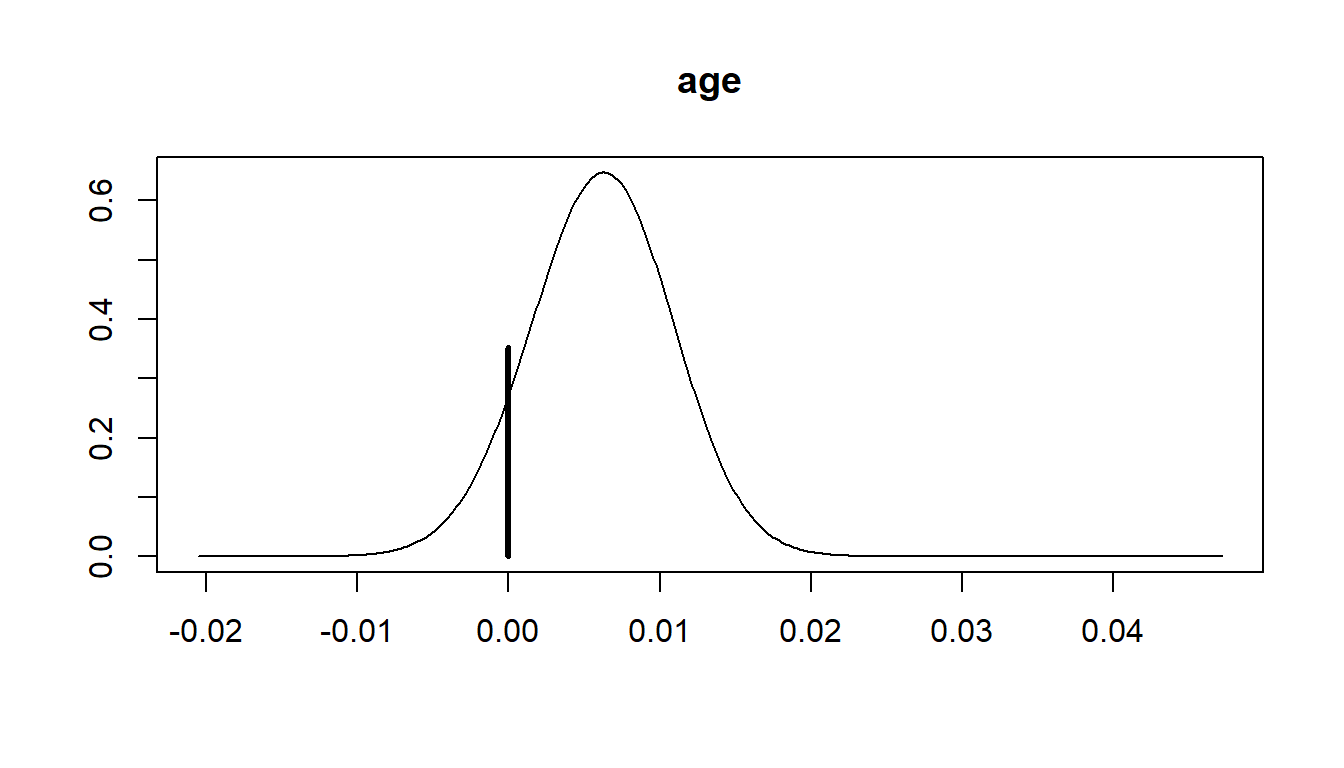## Prediction

A key advantage of Bayesian statistics is prediction and the probabilistic interpretation of predictions. Much of Bayesian prediction is done using simulation techniques, some of which was discussed near the end of this module. This is often applied in regression modeling, although we’ll work through an example with just an intercept term.

Suppose you observe four numerical observations of $$y$$, which are 2, 2, 0 and 0 respectively with sample mean $$\bar{y} = 1$$ and sample variance $$s^2 = 4/3$$. Assuming that $$y \sim N(\mu, \sigma^2)$$, under the reference prior $$p(\mu,\sigma^2) \propto 1/\sigma^2$$, our posterior becomes

$\mu|\sigma^2, y \sim N(1, \sigma^2/4)$ which is centered at the sample mean and $1/\sigma^2, y \sim Gamma(\alpha = 3/2,\beta = 4/2)$ where $$\alpha = (n - 1)/2$$ and $$\beta = s^2 (n-1)/2 = 2$$.

To obtain the predictive distribution for $$y_5$$, we can first simulate $$\sigma^2$$ from its posterior and then $$\mu$$ followed by $$y_5$$. Our draws of $$y_5$$ will be from the posterior predictive distribution for a new observation. The example below draws 100,000 times from the posterior predictive distribution of $$y_5$$.

set.seed(314)
N = 100000
phi = rgamma(N,3/2,2)
sigma2 = 1/phi
mu = rnorm(N, 1, sqrt(sigma2/4))
y_5 = rnorm(N, mu, sqrt(sigma2))

We can view an estimate of the predictive distribution, by looking at the a smoothed version of the histogram of the simulated data:

hist(y_5, prob=T, breaks=30, xlab=expression(y), main="")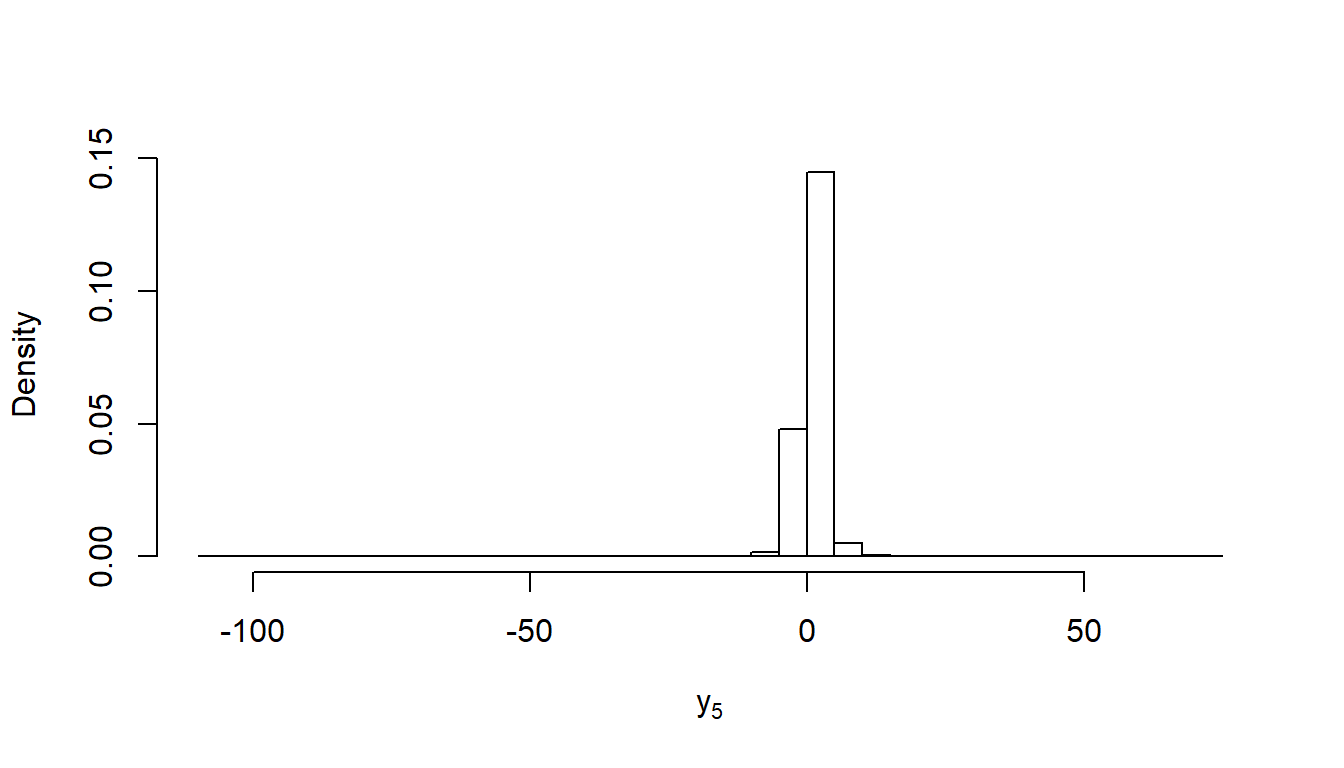A 95% central credible interval for a new observation is the interval (L, U) where $$P(Y_5 < L \mid Y) = .05/2$$ and $$P(Y_5 > U \mid Y) = .05/2)$$. In this case L is the 0.025 quantile and U is the 0.975 quantile. We can obtain those values using the quantile function to find the sample quantiles for 0.025 and 0.975 of $$y_5$$.

1. Estimate a 95% central credible interval for a new observation $$y_5$$
1. (-3.71, 5.73)
2. (-3.11, 5.13)
3. (-1.18, 3.19)
# type your code for Question 9 here, and Knit
format(quantile(y_5, probs = c(.025, .975)), digits=2, nsmal=2)
##    2.5%   97.5%
## "-3.11" " 5.13"

Exercise: In the simple example above, it is possible to use integration to calculate the posterior predictive analytically. In this case, it is a scaled $$t$$ distribution with 3 degrees of freedom $$(n - 1)$$ with mean $$1$$ and scale = 5/3 ($$s^2(1 + 1/n)$$). Plot the empirical density of $$y$$ alongside the actual density of the t-distribution. How do they compare?

# type your code for the Exercise here, and Knit
dat <- data.frame(y = rt(n = 4, df = 3)*5/3)
set.seed(314)
N = 100000
t_d = rt(N, df = 3, ncp = 1)
hist(y_5, prob=T, breaks=30, xlab=expression(y), main="")hist(t_d, prob=T, breaks=30, xlab="t_d", main="")